# 1. Multiscalar Territorial Analysis for Policy Study

The aim of this case-study consists in exploring functions proposed by the MTA package in a structured path of investigation (following the logic proposed by HyperAtlas) by associating MTA functions with relevant maps and plots.
In this vignette, we will investigate the concrete example of income inequalities in the Metropolis of Greater Paris (Métropole du Grand Paris).
Several elements must be considered: the study area, the territorial hierarchy and the selected indicator.

• Metropolis of Greater Paris (MGP) : a study area making sense in a political context.
The MGP has been created the 1st January 2016 after a prefiguration mission and aims at finding solutions to improve and develop the potential of the Paris metropolitan area to be more present at international level (following the initiatives that took place in London, New-York or Tokyo). The official website (last visit: 2016-03) of this infrastructure provides all the details of this new level of governance. Namely, this spatial planning project proposes to “develop a stronger solidarity between territories, reduce territorial inequalities and propose a re-equilibrium in term of access to household, employment, training, services and equipments”. For this emerging zoning of spatial planning, the need for indicators and analysis of territorial inequalities is high. The MTA methodology seems to be particularly adapted to propose territorial evidences in this context.

• From the IRIS to the MGP, a lot of hierarchical territorial divisions are available.
The lowest territorial level existing in France associated with public statistical information is IRIS (Ilots Regroupés pour l’Information Statistique). The reference size targeted for each IRIS is 2000 inhabitants. Each commune over 10 000 inhabitants and a high proportion of communes between 5 000 and 10 000 inhabitants are split in IRIS. It is possible to merge these IRIS in communes (36 000 territorial units in France). These communes can also be merged in Établissements Publics Territoriaux or in Départements. Each territorial context has specific competences in spatial planning term. Thus, the MGP is constituted by a large number of territorial divisions. It allows the calculation of a high number of deviations according to these territorial contexts. This analysis is mainly focused on communes and Établissements Publics Territoriaux (EPT) but could be extended to other territorial levels.

• Indicators relevant at this scale of analysis.
The chosen indicator (average income tax reference per households) is one of the key drivers of spatial planning policies at local level in France. It is one of the indicators considered relevant for city policy (Politique de la Ville).

This vignette proposes some concrete ouptuts for improving the knowledge on income inequalities and proposing solutions for a better geographical repartition of wealth in the MGP area. Relevant statistics will be computed using MTA functionalities and plotted on graphics and maps.

# 2. Dataset and Complementary Packages

• com.spdf and ept.spdf: 2 SpatialPolygonsDataFrame. com.spdf is a SpatialPolygonsDataFrame of the 150 MGP communes. ept.spdf is a SpatialPolygonsDataFrame of 12 MGP EPTs. (see ?com.spdf and ?ept.spdf)

• com: a data frame of data on the MGP communes. It includes communes identifiers (DEPCOM), communes names (LIBCOM), identifiers and names of the EPTs of belonging of each commune (EPT and LIBEPT), identifier of the departement of belonging of each commmune (DEP) and the statistical indicators for the multiscalar inequality analysis, the numerator (amount of income tax references in Euros, INC) and the denominator (number of tax households, TH) (see ?com).

We use 2 additional and complementary packages in this vignette: cartography for thematic mapping purposes and ineq for computing inequality indexes and Lorenz Curve Plot.

``````# load packages
library(MTA)
library(cartography)
library(sp)
library(ineq)
library(reshape2)

data("GrandParisMetropole", package = "MTA")
# set row names to communes names
row.names(com) <- com\$LIBCOM``````

# 3. Context Maps

Context maps are useful to begin the MTA. They highlight the territorial organisation of the study area and provide some first insights regarding the spatial patterns introduced by the indicator used for the analysis.

## 3.1 Study Area

``````# set margins
par(mar = c(0,0,1.2,0))

# label management
com\$LIBEPT2 <- com\$LIBEPT
com[com\$LIBEPT == "Val de Bievres - Seine Amond - Grand Orly", "LIBEPT2"] <-
"Val de Bievres -\nSeine Amond - Grand Orly"
com[com\$LIBEPT == "Plaine Centrale - Haut Val-de-Marne - Plateau Briard", "LIBEPT2"] <-
"Plaine Centrale -\nHaut Val-de-Marne - Plateau Briard"
com[com\$LIBEPT == "Association des Communes de l'Est Parisien", "LIBEPT2"] <-
"Association des Communes\nde l'Est Parisien"

# label order
epts <- c("Paris",
"Est Ensemble",
"Grand-Paris Est",
"Territoire des aeroports",
"Plaine Commune",
"Boucle Nord 92",
"La Defense",
"Grand Paris Sud Ouest",
"Sud Hauts-de-Seine",
"Val de Bievres -\nSeine Amond - Grand Orly",
"Plaine Centrale -\nHaut Val-de-Marne - Plateau Briard",
"Association des Communes\nde l'Est Parisien")

# colors
cols <- c("purple", carto.pal("blue.pal", 8)[c(1,3,6,8)],
carto.pal("green.pal", 8)[c(1,3,6,8)],
carto.pal("red.pal", 8)[c(3,5,8)])

# zoning
typoLayer(spdf = com.spdf, df = com,
var="LIBEPT2", legend.values.order = epts,
legend.pos = "left",
col = cols,
legend.title.txt = "EPT")

# layout
layoutLayer(title = "Territorial Zoning of the MGP",
sources = "GEOFLA® 2015 v2.1, Apur",
author = "RIATE, 2016",
scale = 5,
frame = TRUE,
col = "black",
coltitle = "white",
south = FALSE)``````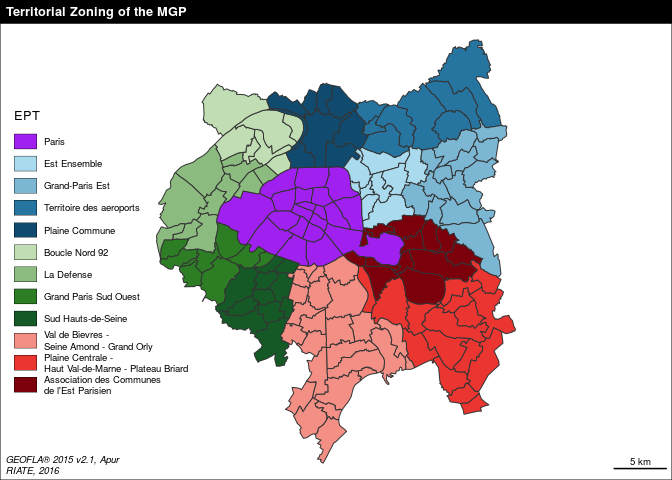The 150 municipalities of the MGP are grouped in 12 intermediate zonings: the Établissements Publics Territoriaux (EPT). This territorial zoning respect approximately the delineation of the départements : the EPTs of Seine-Saint-Denis are displayed in blue palette; the EPT of Paris is displayed in purple; the EPTs of Hauts-de-Seine are displayed in green palette (it includes also one municipality of Val-d’Oise in the North-West of Boucle Nord 92) and the EPTs of Val-de-Marne are displayed in red palette (it includes also 6 municipalities in the south part of Val-de-Bièvres EPT).

## 3.2 Numerator (amount of income tax reference) and Denominator (number of tax households)

First it is interesting to plot on maps the different statistical dimensions of the indicator we are interested in: the numerator (total amount of income tax references), the denominator (number of tax households) and the ratio (average income per tax households).

``````# layout
par(mfrow = c(1,2), mar = c(0,0,1.2,0))

# numerator map
com\$INCM <- com\$INC / 1000000
plot(com.spdf,  col = "peachpuff",  border = "grey20",  lwd = 0.2)
propSymbolsLayer(spdf = com.spdf, df = com,
var = "INCM",
symbols = "circle", col =  "#F6533A",
inches = 0.15,
border = "#25252570",
legend.pos = "topleft", legend.values.rnd = 0,
legend.title.txt = "Amount of income taxe reference\n(millions of euros)",
legend.style = "c")
# layout
layoutLayer(title = "Numerator - Amount of income tax reference",
sources = "GEOFLA® 2015 v2.1, Apur, impots.gouv.fr",
author = "RIATE, 2016",
scale = NULL,
frame = FALSE,
col = "black",
coltitle = "white")

# denominator map
plot(com.spdf, col = "peachpuff",border = "grey20",lwd=0.2)
propSymbolsLayer(spdf = com.spdf, df = com,
var = "TH",
symbols = "circle", col =  "#515FAA",
border = "#25252570",
inches = 0.15,
legend.pos = "topleft", legend.values.rnd = -2,
legend.title.txt = "Number of tax households",
legend.style = "c")

# layout
layoutLayer(title = "Denominator - Tax households",
sources = "",
author = "",
scale = 5,
frame = FALSE,
col = "black",
coltitle = "white")``````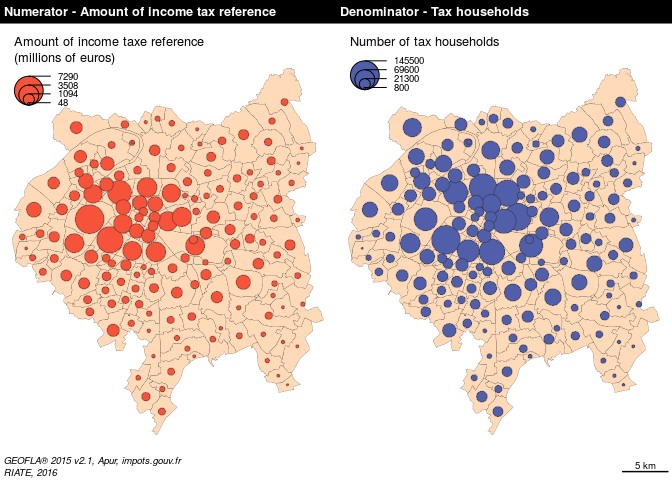Without surprise, the highest amounts of tax households and income are located in the central area of the MGP (Paris arrondissements). That being said, these two maps suggest an inequal repartition of income in regard to the repartition of population in Paris suburbs.

## 3.3 Ratio (average amount of income tax reference per households)

``````# margins
par(mar = c(0,0,1.2,0))

# ratio
com\$ratio <- com\$INC / com\$TH

# ratio map
choroLayer(spdf = com.spdf,
df = com,
var = "ratio",
breaks = c(min(com\$ratio,na.rm=TRUE),20000,
30000,40000,50000,60000,
max(com\$ratio,na.rm=TRUE)),
col = carto.pal(pal1 = "red.pal", n1 = 6),
border = "grey20",
lwd=0.2,
legend.pos = "topleft",
legend.title.txt = "Average amount of income tax\nreference per households\n(in euros)",
legend.values.rnd = 0)

# EPT borders

# layout
layoutLayer(title = "Ratio - Income per tax households, 2013",
sources = "GEOFLA® 2015 v2.1, Apur, impots.gouv.fr",
author = "RIATE, 2016",
scale = NULL,
frame = FALSE,
col = "black",
coltitle = "white")``````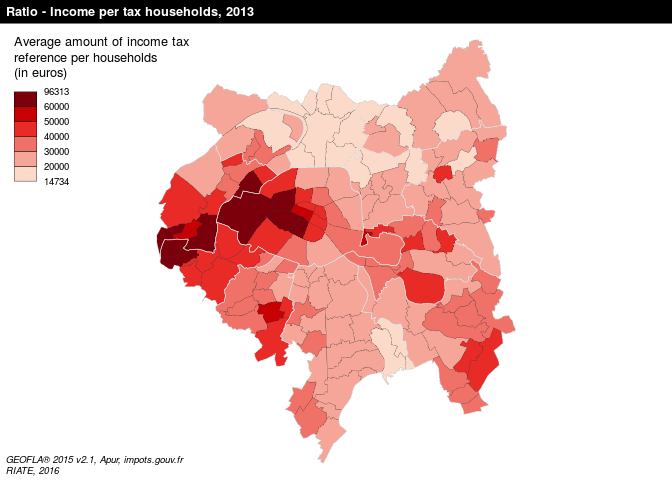The MGP area is characterised by high income inequalities. For the 150 communes of this area, the values extend from 14 730 (La Courneuve) to 96 310 euros (Paris, 7th arrondissement). 53 municipalities of the MGP area (35 % of the communes) are below the French average, i.e. 25 660 euros. The lagging households are mainly concentrated into the north part of MGP area. Highest values are concentrated in the Western part of Paris and its suburbs.

# 4. Intoducing the MTA Functions

## 4.1 General, territorial and spatial deviations

MTA package introduces three contexts to monitor territorial inequalities: the general deviation, the territorial deviation and the spatial deviation.

The global deviation is dedicated to the analysis of inequalities using a value of reference. In this example the global deviation refers to the inequalities existing between each commune in regard to the whole Metropole du Grand Paris value.

The territorial deviation consists in measuring the inequalities existing for each basic territorial unit in regard to an intermediate territorial level of reference. In this case-study, for each basic territorial unit (Communes of the MGP in this case), it implies to include beforehand in the input dataset a territorial a factor describing the intermediate territorial belonging (departements or EPT). It allows to measure for each commune the deviation existing as regards to their EPT or departement of belonging.

The spatial deviation is a measure of inequalities taking into account the neighbourhood as a reference. It allows to measure the deviation existing between basic territorial units using three possible parameters : the territorial contiguity (order 1, 2 or n), the spatial neighbourhood (territorial units located at less than X kilometers as the crow flies) or functional distances (territorial units located at less than Y minutes by road for instance). In MTA, territorial contiguity and spatial neighbourhood measures are calculated directly from the spatialdataframe. Functional distances must be uploaded separately through a dataframe structured with the following fields : id1, id2, distance measure. For this case-study, the contiguity criteria (order 1) has been retained for the calculation of the spatial deviation. It gives a good proxy of proximity relationships according to the size and the homogeneity of the communes of the MGP area. Other measures could be also adapted, such as time-distances by road (communes located at less than 15 minutes by car) or by public transport.

## 4.2 Relative and absolute deviations

In MTA, two methods are implemented to measure statistical differences to a given context of reference: the relative deviation and the absolute deviation. In MTA, each indicator is considered as a ratio defined by a numerator (GDP for instance) divided by a denominator (population for instance).

The relative deviation states the position of each region as regard to a context of reference expressed in index 100. It is based on the following calculation: Relative deviation (Region i) = 100 * ((Numerator(Region i)/Denominator(Region i)) /(reference ratio)) Territorial units characterised by a context of reference below index 100 are under the average of a given context of reference, and reciprocally.

The absolute deviation specifies which process of redistribution should be realised in absolute terms in order to achieve perfect equi-repartitition of the ratio of reference in the global, the territorial or the spatial context. It is calculated as below: Absoute deviation (Region i) = Numerator (Region i) - (reference ratio * denominator (Region i)) It examines how much amount of the numerator should be moved in order to reach equi-repartition, for each territorial unit, taking into account as a reference the selected deviation context value. More generally, absolute deviation must be considered as a statistical tool to discuss on the amplitude of existing territorial inequalities in absolute terms. It is obvious that reaching a perfect equilibrium between territorial units is highly sensitive and is scarcely a policy objective itself. It is neverthess interesting to consider the amount of money or population affected by the inequality to have in hand a concrete material for leading discussions on this delicate spatial planning issue.

## 4.3 Cartography of relative and absolute deviations

In this vignette, color palette proposed for displaying on map the relative deviations are the ones suggested by the HyperAtlas tool (blue palette = under the average; red palette = above the average). But other diverging palettes (green/red, etc.) could be also be used for displaying MTA results on maps.
Absolute deviations are highlighted using proportional circles on maps. It examines which amount of the numerator should be moved to the poorest communes to reach equi-repartition. Circles displayed in a red palette means that the territorial unit have to contribute a given amount of numerator to achieve the equilibrium in a given context; and reciprocally circles displayed in a blue palette means that the territorial unit have to receive a given amount of numerator to achieve perfect convergence.

In this example, we have decided to merge in a same map the relative deviation (colour of the circles) and the absolute deviation (size of the circles) to be more synthetic. In HyperAtlas tool, this map is split in two maps (one for the relative and one for the absolute deviation). It is quite easy to reproduce these maps using the functionalities of the cartography package.

## 4.4 Synthesis

The analysis of the three deviations provides generally a high number of information, difficult to synthesise. The synthesis functions helps to summarise values of the global, territorial and spatial deviations and allow to answer to some basic and interesting questions:

• Which territorial units are positioned in a favourable situation for the three deviations?
• Which territorial units are positioned in a lagging situation fot the three deviations?
• Which territorial units are positioned in a contradictory situation? It means that a territorial unit is situated above a given threshold for a context and under the same threshold for the other contexts. This situation is especially interesting from a social and policy points of view (NIMBY phenomena, characterizating the opposition by residents to a proposal for a new development because it is close to them).

# 5. Global deviation

This part proposes some graphical outputs helping to have an idea regarding inequalities existing at the global level. In this case, for all the study area: the Metropole du Grand Paris.

## 5.1 Global deviation and theoretical redistribution

The code below takes in entry the numerator (INC) and the denominator (TH) and returns the global deviation indicators (relative and absolute). These indicators are afterwards associated with the input SpatialDataFrame (com.spdf) for displaying on map this deviation.

``````# general relative deviation
com\$gdevrel <- gdev(x = com,
var1 = "INC",
var2 = "TH",
type = "rel")

# general absolute deviation
com\$gdevabs <- gdev(x = com,
var1 = "INC",
var2 = "TH",
type = "abs")

# general deviation in million Euros
com\$gdevabsmil <- com\$gdevabs / 1000000

# margins
par(mar = c(0,0,1.2,0))

# Plot territories
plot(com.spdf, col = "grey70", border = "#EDEDED", lwd = 0.25)
plot(ept.spdf, border = "#1A1A19", lwd = 1, add = TRUE)

# Global deviation (relative and absolute) cartography
propSymbolsChoroLayer(spdf = com.spdf, df = com,legend.var.values.rnd = 4,
var = "gdevabsmil", var2 = "gdevrel",
inches = 0.3,
col = carto.pal(pal1 = "blue.pal", n1 = 3,
pal2 = "wine.pal", n2 = 3),
breaks = c(min(com\$gdevrel,na.rm=TRUE),
75,90,100,111,133,
max(com\$gdevrel,na.rm=TRUE)),
border = "#f0f0f0",
lwd = 0.25,
legend.var.pos = "left", legend.var2.pos = "topleft",
legend.var.title.txt = "Redistribution (Million euros)",
legend.var2.title.txt = "Deviation to the global context (100 = Metropole du Grand Paris average)",
legend.var.style = "e")

layoutLayer(title = "Global deviation - Tax income per households",
sources = "GEOFLA® 2015 v2.1, Apur, impots.gouv.fr",
author = "RIATE, 2016",
scale = NULL,
frame = FALSE,
col = "black",
coltitle = "white")``````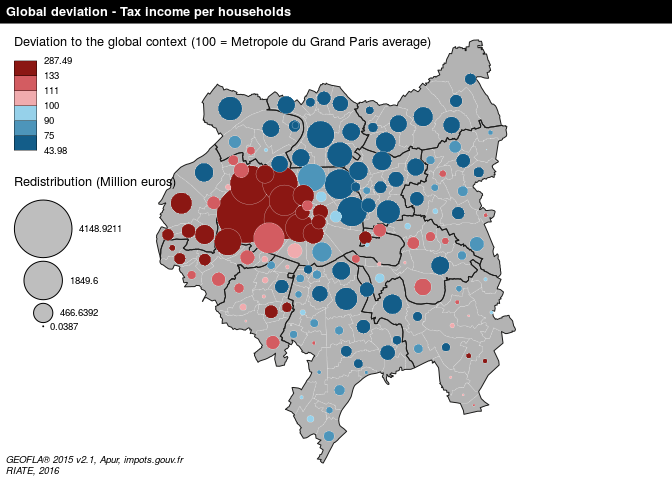The resulting map highlights strong statistical differences of income earning in the MGP area (colour of the circles). It is firstly interesting to know that all the communes of the EPT of Plaine Commune, Territoire des aéroports and Est Ensemble are below the average of the MGP area (33 501 euros per households). For the territories of Val de Bièvres and Grand-Paris Est, only three communes are above the average of the study area. Reversely, all the communes of the Grand-Paris-Sud-Ouest EPT are largely above the average of the MGP area. For the other EPTs the situation is mixed, depending of the municipalities.

This map and these tables highlight the communes which may have to contribute / receive the highest to ensure a perfect equi-repartition in the context of the MGP (size of the circles). In this example, the 7th Arrondissement of Paris is the municipality which should contribute the most, all things being equal to its income tax level (1,987 billion Euros of income transfer, 65 % of the total amount of tax income in this municipality). Neuilly-sur-Seine (third position in absolute terms) should transfer 1,9 billion Euros to the lagging communes of the MGP area. It corresponds to 62,87% of the total amount of tax income declared in this municipality.

In the other side of the redistribution, La Courneuve should receive 402 million Euros from the wealthiest communes (127 % of the current tax income declared). Aubervilliers should receive 793 million Euros, which represents 124 % of the current tax income in this commune.

The code below allows to order the communes that have to receive / contribue the most in relative term in the global context to ensure a perfect equilibrium of resources.

``````# general deviation - Top 10 of the potential contributors in regard
# to their total amount of income
com\$gdevabsPerc <- com\$gdevabs / com\$INC * 100
com <- com[order(com\$gdevabsPerc, decreasing = TRUE), ]
com[1:10, c("gdevabsmil","gdevabsPerc")]``````
``````##                          gdevabsmil gdevabsPerc
## Paris 7e Arrondissement  1987.18261    65.21643
## Marnes-la-Coquette         46.58689    63.53843
## Neuilly-sur-Seine        1900.79972    62.87958
## Paris 8e Arrondissement  1167.45176    59.37545
## Paris 16e Arrondissement 4148.92114    56.91599
## Paris 6e Arrondissement  1030.25933    55.83634
## Vaucresson                174.81548    54.71988
## Saint-Cloud               473.60724    48.07040
## Ville-d'Avray             165.60016    46.23143
## Garches                   246.70718    43.19362``````
``````# general deviation - Top 10 of the potential receivers in regard to
# their total amount of income
com <- com[order(com\$gdevabsPerc, decreasing = FALSE), ]
com[1:10,c("gdevabsmil","gdevabsPerc")]``````
``````##                          gdevabsmil gdevabsPerc
## La Courneuve              -402.2023  -127.37566
## Aubervilliers             -793.0032  -124.08051
## Clichy-sous-Bois          -243.2493  -114.90485
## Bobigny                   -465.2779  -108.01736
## Stains                    -313.7436  -105.88273
## Villetaneuse              -104.6940   -99.97363
## Saint-Denis               -941.8914   -93.24860
## Pierrefitte-sur-Seine     -237.5380   -92.02924
## Villeneuve-Saint-Georges  -279.8035   -85.87404
## Dugny                      -81.3603   -83.81888``````

## 5.2 Lorenz Curve and inequality indexes

The library ineq proposes some functions useful for depicting global inequalities existing in a study area. The Lorenz-curve was developed first by Max O. Lorenz in 1905 as a graphical representation of income distribution. The Lorenz Curve function takes in entry the numerator and the denominator and returns a Lorenz Curve plot; inequality indexes take in entry the ratio (numerator / denominator) and returns econometric indexes of inequality.

``````par(cex.lab=1)
par(cex.axis=0.75)
par(mar=c(4,4,2,2))

# Lorenz Curve
Lc <- Lc (com\$INC, n=com\$TH)
plot(Lc,
ylab = "proportion of numerator (total tax income)",
xaxt = 'n',
xlab = "proportion of denominator (households)",
yaxt = 'n')

seq<-seq(0,1,0.1)
axis(side = 1, at = seq,labels = T)
axis(side = 2, at = seq, labels = T)

grid(10, 10, lwd = 1)``````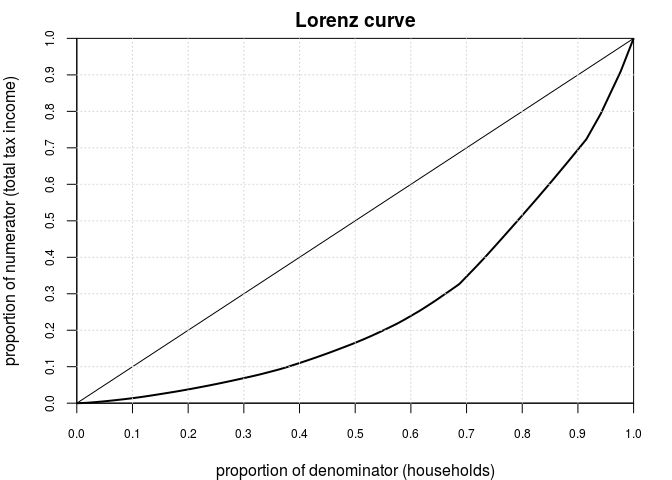``````# Inequality indexes
Gini<-ineq(com\$ratio)
Coeff.Var<-var.coeff(com\$ratio, square = FALSE, na.rm = TRUE)
Gini``````
``##  0.2299973``
``Coeff.Var``
``##  0.4552654``

The curve depicts on its horizontal axis a defined population – e.g., all households – broken down into deciles and ordered from, from left to right on the horizontal axis, from the lower tax income per household to the higher. On the vertical axis of the Lorenz curve is shown the cumulative percentage of tax income.

This plot reveals these following configurations as regard to social household repartition in MGP: * 50 % of the households earnes less than 20 % of the total income. * 50 % of the total income is held by less than 22 % of households.

The analysis of Gini and variation coefficient give a global overview of the degree of inequality. The Gini index is comprised between 0 (equirepartition) and 1 (maximal concentration). But more interesting is analysis of the evolution of these indexes over the time (more equality ? less inequality ?)

# 6. Territorial deviation

This part proposes some graphical representations helping to have an idea regarding inequalities existing at territorial level. In this case, as regard to the average of each Etablissement Public Territoriaux (EPT).

## 6.1 Territorial deviation and theoretical redistribution

The code below takes in entry the numerator (INC) and the denominator (TH) and returns the territorial deviation indicators (relative and absolute). These indicators are afterwards associated with the input SpatialDataFrame (com.spdf) for displaying on map this deviation.

``````# Territorial relative deviation calculation
com\$mdevrel <- tdev(x = com,
var1 = "INC",
var2 = "TH",
type = "rel",
key = "LIBEPT")

# Territorial absolute deviation calculation
com\$mdevabs <- tdev(x = com,
var1 = "INC",
var2 = "TH",
type = "abs",
key = "LIBEPT")

# Territorial deviation in million Euros
com\$mdevabsmil <- com\$mdevabs / 1000000

# Cartography
# Plot layout
par(mfrow = c(1,1), mar = c(0,0,1.2,0))
layoutLayer(title = "Territorial deviation - Tax income per households, 2013",
sources = "Data source : DGFiP, 2016",
author = "Author : RIATE, 2016",
scale = 5,
frame = TRUE,
col = "black",
coltitle = "white",
bg = "#FFFFFF",
south = FALSE,
extent = com.spdf)

# Plot territories
plot(com.spdf, col = "grey70", border="#EDEDED",lwd=0.25,add=T)
plot(ept.spdf, border = "#1A1A19", lwd = 1, add = T)

# Territorial deviation (relative and absolute) cartography
propSymbolsChoroLayer(spdf = com.spdf, df = com,
var = "mdevabsmil", var2 = "mdevrel",
inches = 0.3,
col = carto.pal(pal1 = "blue.pal", n1 = 3,
pal2 = "wine.pal", n2 = 3),
breaks = c(min(com\$mdevrel,na.rm=TRUE),
75,90,100,111,133,
max(com\$mdevrel,na.rm=TRUE)),
border = "#f0f0f0",
lwd = 0.25,
legend.var.pos = "left", legend.var2.pos = "topleft",
legend.var.title.txt = "Redistribution (Million euros)",
legend.var2.title.txt = "Deviation to the territorial context (100 = Etablissement public territoriaux average)",
legend.var.style = "e")``````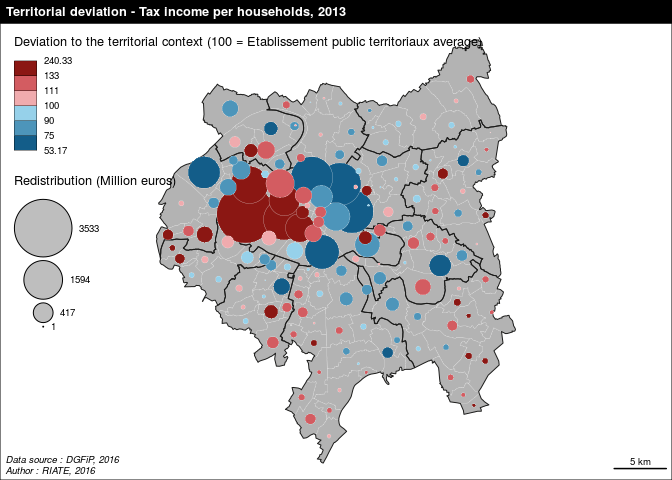The map highlight important statistical differences in each EPT in relative terms. The strongest differences in relative terms are located in Paris (opposition between the eastern part and the western part of this EPT) and in the Plaine centrale - Haut Val de Marne EPT (opposition beween the poorest municipalities located near Paris and the ones located in the periphery). Globally, the richest and the poorest EPT (Grand Paris Sud Ouest / Plaine Commune and Territoires des aéroports du Nord Ouest) appear relatively homogeneous statistically. In other EPTs, one municipality appears largely above the average of their EPT of belonging. It is the case in Est Ensemble (Les Lilas), Boucle-Nord 92 (Bois-Colombes), Sud Hauts-de-Seine (Sceaux)

The circles highlight the communes which may have to contribute (red palette) / receive (blue palette) the highest to ensure a perfect equilibrium of income per housold for each Etablissement Public Territoriaux. The 7th Arrondissement of Paris is the commune which should contribute the most to the poorest communes of Paris as regards to the amount of income available in this commune (1,779 billion Euros of income transfer, 58 % of the amount of income in this commune). Marnes-la-coquette (second position) should transfer 38 million Euros to the poorest communes of its EPT of belonging (La Défense). It is relatively low as regards to the 7th arrondissement of Paris, but it corresponds to 52 % of the total amount of income available in this commune.

From the other side of the redistribution, Nanterre, Clichy-sous-bois and the 19th arrondissement of Paris should receive respectivally 1088, 143 and 1926 million euros from the richest communes of their EPT of belonging. It represents respectively 88 %, 68 % and 65 % of the total amount of earned income of their households.The highest redistribution for this study area stands for the 20th arrondissement of Paris (1,926 billion euros, 59 % of its total amount of income).

``````# Territorial deviation - Top 10 of the potential contributors as regards to their total amount of income
com\$mdevabsPerc<- com\$mdevabs / com\$INC * 100
com<-com[order(com\$mdevabsPerc, decreasing = TRUE), ]
com[1:10,c("mdevabsmil","mdevabsPerc")]``````
``````##                          mdevabsmil mdevabsPerc
## Paris 7e Arrondissement  1779.21614    58.39127
## Marnes-la-Coquette         37.85668    51.63157
## Paris 8e Arrondissement  1010.71931    51.40419
## Neuilly-sur-Seine        1468.33529    48.57340
## Paris 16e Arrondissement 3532.67330    48.46214
## Paris 6e Arrondissement   870.36502    47.17065
## Santeny                    37.74031    43.38812
## Marolles-en-Brie           47.14636    42.74486
## Vaucresson                119.06443    37.26896
## Le Raincy                 116.35420    35.78738``````
``````# Territorial deviation - Top 10 of the potential receivers as regards to their total amount of income
com<-com[order(com\$mdevabsPerc, decreasing = FALSE), ]
com[1:10,c("mdevabsmil","mdevabsPerc")]``````
``````##                          mdevabsmil mdevabsPerc
## Nanterre                 -1087.5931   -88.08889
## Clichy-sous-Bois          -143.1380   -67.61479
## Paris 19e Arrondissement -1925.8379   -65.44786
## Paris 20e Arrondissement -1958.2712   -59.73389
## Bagneux                   -295.2509   -58.59873
## Paris 18e Arrondissement -1864.1104   -53.46040
## Champigny-sur-Marne       -509.8546   -49.20335
## Gennevilliers             -197.9141   -43.87097
## Villeneuve-Saint-Georges  -123.2206   -37.81744
## Paris 13e Arrondissement -1241.4815   -36.30654``````

## 6.2 Box-plot by Etablissement Public Territorial

Another way to explore characteristics of the territorial deviation consists in analysing the statistical dispersion (general deviation) by intermediate level (EPT in this case). The best suited graphical representation for this kind of analysis is certainly the boxplot.

The code below takes in entry the general deviation calculated above and the intermediate levels included in the input dataset (com). It returns a boxplot displaying the statistical parameters (median, mean, 1st and 3rd quartiles, range, minimum and maximum, extraordinary values) allowing to observe the statistical dispersion existing for each intermediate zoning (in this case each EPT). To ease the interpretation and the synthesis of the plot, boxplots are ordered by mean values for each intermediate levels. Moreover, the width of the bars are proportional to the number of territorial units included in each intermediate zoning.

``````# Layout parameters
par(cex.lab=1)
par(cex.axis=0.75)
par(mar=c(4,4,2,2))

# Boxplot ordered by mean
com\$EPT <- with(com, reorder(EPT, gdevrel, mean))

boxplot(com\$gdevrel ~ com\$EPT,
col = carto.pal(pal1 = "wine.pal", n1 = nlevels(com\$EPT)),
ylab = "Global deviation",
varwidth = TRUE,
range = 1,
outline = TRUE,
las = 1)

abline (h = seq(40,290,10), col = "gray70", lwd = 0.25, lty = 3)
abline (h = seq(50,250,50), col = "gray0", lwd = 1, lty = 1)

par(new=TRUE)

boxplot(com\$gdevrel ~ com\$EPT,
col = carto.pal(pal1 = "wine.pal", n1 = nlevels(com\$EPT)),
ylab = "Global deviation",
varwidth = TRUE,
range = 1,
outline = TRUE,
las = 1)

# Plot mean values
xi<- tapply(com\$gdevrel,com\$EPT,mean)
points(xi,col="#7C0000",pch=19)

# Legend for the boxplot
com\$LIBEPT <- as.factor(com\$LIBEPT)

legend("topleft",
legend = levels(com\$LIBEPT),
pch = 20,
col = carto.pal(pal1 = "wine.pal", n1 = nlevels(com\$EPT)),
cex = 0.8,
pt.cex = 1,
title = "Territorial contexts (ordered by mean value of global deviation)")``````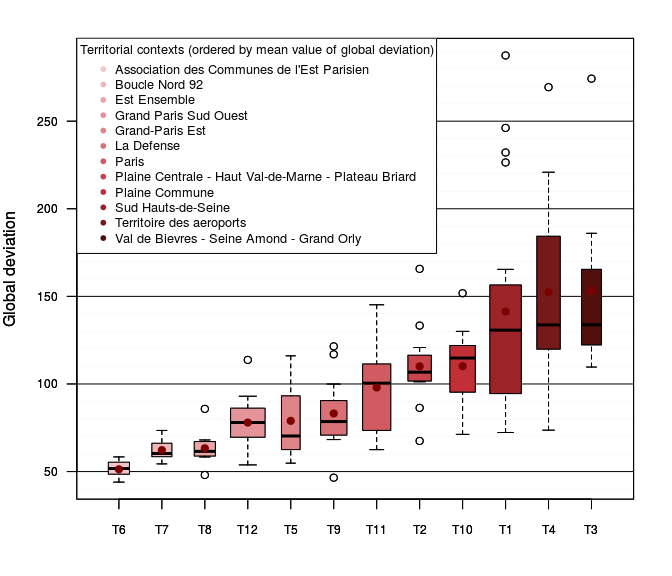This plot highlights the statistical dispersion existing in each Etablissement Public Territoriaux. It completes the analyis proposed in the previous map. This boxplot delivers several learnings: Firstly, it confirms globally that wealthier the EPT is, larger the statistical differences between the poorest and the wealthiest territorial units are. In this perspective, Plaine Commune and Territoire des Aeroports (T6 and T7) are quite homogeneous (all the communes of these territories are lagging). On the reverse, the arrondissements of Paris are characterised by strong diffenrences between minimum (index 71) and maximum values (index 90). The same is true with La Defense and Grand Paris Sud Ouest (T4 and T3) with important interquartle values. Secondly, extraordinary values (dots out of the box) concerns poor and wealthy EPT. But for this study area outliers mainly concern maximum values, especially for Paris, La Défense, Grand Paris Sud Ouest, ACEP). Finally, it is interesting to note that Est Ensemble, Grand Paris Est and Sud Hauts-de-Seine (EPT T8, T9 and T2) include communes with very low average income per household, since they are characterised by outliers in the low values.

# 7. Spatial deviation

This part proposes some graphical representations helping to have an idea regarding inequalities existing in a local (or spatial) context. In this case, as regard to the average of contiguous territorial units (contiguity order 1).

## 7.1 Spatial deviation and theoretical redistribution

The code below takes in entry the numerator (INC) and the denominator (TH) and returns the spatial deviation indicators (relative and absolute). These indicators are afterwards associated with the input SpatialDataFrame (com.spdf) for displaying on map this deviation.

``````par(mar=c(2,4,0,0))

# Spatial relative deviation calculation
com\$ldevrel <- sdev(spdf = com.spdf,
x = com,
spdfid = "DEPCOM",
xid = "DEPCOM",
var1 = "INC",
var2 = "TH",
order = 1,
type = "rel")

# Spatial absolute deviation calculation
com\$ldevabs <- sdev(spdf = com.spdf,
x = com,
spdfid = "DEPCOM",
xid = "DEPCOM",
var1 = "INC",
var2 = "TH",
order = 1,
type = "abs")

# Spatial deviation in million Euros
com\$ldevabsmil <- com\$ldevabs / 1000000

# Cartography
# Plot layout
par(mfrow = c(1,1), mar = c(0,0,1.2,0))
layoutLayer(title = "Spatial deviation - Tax income per households, 2013",
sources = "Data source : DGFiP, 2016",
author = "Author : RIATE, 2016",
scale = 5,
frame = TRUE,
col = "black",
coltitle = "white",
bg = "#FFFFFF",
south = FALSE,
extent = com.spdf)

# Plot territories
plot(com.spdf, col = "grey70", border="#EDEDED",lwd=0.25,add=T)

# Territorial deviation (relative and absolute) cartography
propSymbolsChoroLayer(spdf = com.spdf, df = com,
var = "ldevabsmil", var2 = "ldevrel",